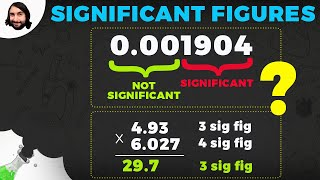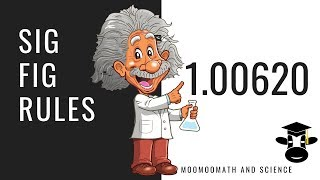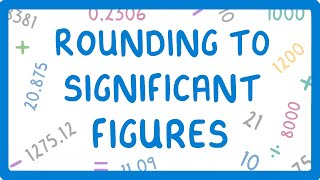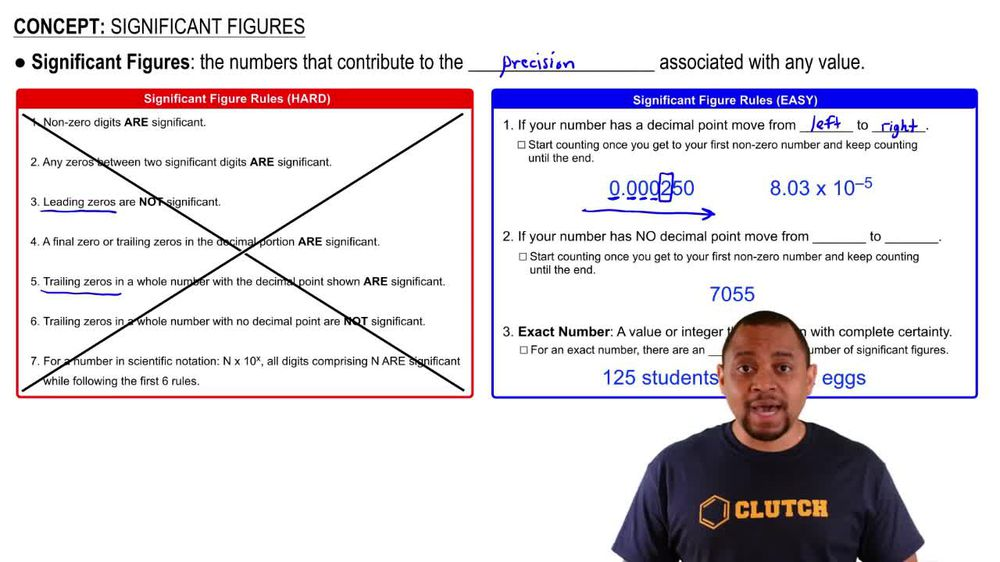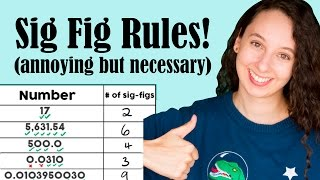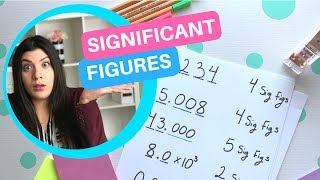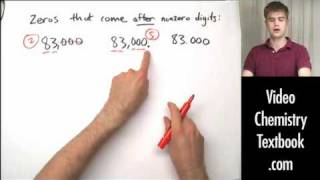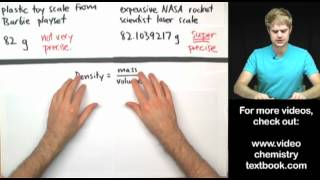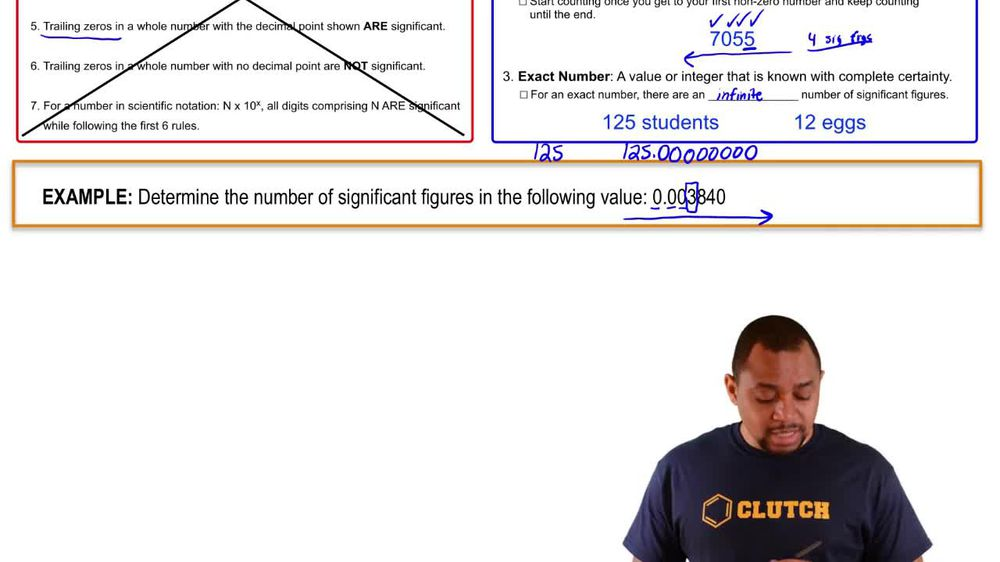Start typing, then use the up and down arrows to select an option from the list.
1. 1. Intro to General Chemistry2. Significant Figures
Problem

# Round off the following quantities to the number of signifi-cant figures indicated in parentheses. (c) 4.995 * 103 cm (3)

Relevant Solution2m
Play a video:
Hi everyone for this question we need to round each of the following 22 significant figures. So let's go ahead and get started for part a we have 32,324 the first thing we need to do is convert this to a decimal. So let's do that by putting a decimal here and moving it to the left 1234 units. So when we do that we get 3.2 and we can raise everything else to a power. So this is going to be times 10 to the four For part B We have 2. times 10 to the 5th. So this is already in a decimal which is great. So let's go ahead and take a look at this zero here because it communicates a level of uncertainty. And so we need to look at the next digit which is a five. And when we have a five we can either increase our zero by one or leave it unchanged. So let's go ahead and increase it by one. And when we do that we will get 2.1 which is two significant figures and will raise everything else to a power. So this is going to be times 10 to the fifth for part C it is in decimal but we need to make it to two decimal places. So let's go ahead and move that decimal to the left. And when we do that we move it 12345 units to the left and when we do that we get 4.5 and we can raise everything else to a power. So that's going to be times 10 to the fifth. And for our last one we have a really long decimal. Now we need to move our decimal place to the right so that we can have two significant figures. So we're going to move this 1, 2, 3, 45, 6, 7, 8, 9, 10, 11, 12, 13. So this is going to move 13 places To the right. And so we'll get 9.3 and we'll raise this to a decimal. And because raise this to a power and because we move to the right, this is going to be a negative power. So this is going to be times 10 to the -13. So these are our final answers, rounded to two significant figures. And that is the end of this problem. I hope this was helpful.